### Name gender over time

February 23rd, 2016
There are various gendered patterns you hear people talk about with names, one of which is that names go from male to female but not the other way around. After a friend put up a challenge to find even a single name that went the other way, I decided to look into the data.

In the US, the Social Security Admninistration provides a list of all names that at least five social security card holders have had, broken down by year and gender. I looked at this list and found all the names that had a decade where they were 60%+ one gender and then a later decade where they were 60%+ the other gender. For example, 86% of babies named "Gale" were male in 1920s, but by the 1950s 81% were female: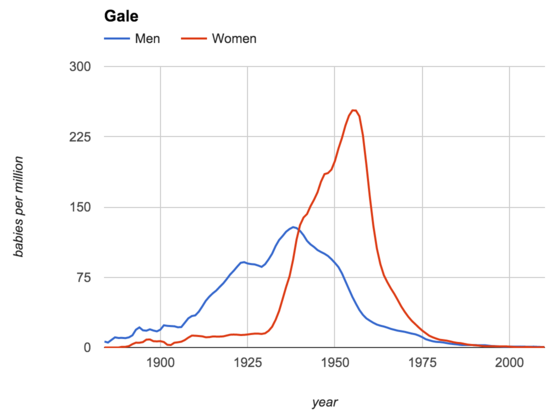Overall, I found 62 popular male-to-female names and 26 female-to-male ones. What do these look like?

##### Names that moved from male to female
Name Male % (n) Male
Female % (n) Female
Addison 100% (n=170) 1880s 93%(n=51348) 2000s
Allison 100% (n=70) 1880s 100%(n=74418) 1990s
Angel 87% (n=1088) 1930s 60%(n=16816) 1970s
Ariel 67% (n=1477) 1970s 86%(n=28135) 1990s
Ashley 100% (n=80) 1880s 100%(n=301779) 1990s
Aubrey 97% (n=3371) 1950s 95%(n=29506) 2000s
Avery 100% (n=132) 1880s 72%(n=41680) 2000s
Bailey 100% (n=78) 1880s 90%(n=4079) 1980s
Beverly 100% (n=107) 1880s 100%(n=3347) 1990s
Billie 78% (n=123) 1880s 89%(n=9978) 1970s
Blair 100% (n=43) 1880s 67%(n=3562) 1990s
Brook 70% (n=709) 1960s 88%(n=3009) 1990s
Carey 96% (n=144) 1880s 67%(n=5603) 1970s
Courtney 67% (n=628) 1940s 97%(n=113275) 1990s
Dana 67% (n=90) 1880s 91%(n=7930) 2000s
Dee 76% (n=276) 1880s 88%(n=4829) 1960s
Elisha 100% (n=336) 1880s 83%(n=4840) 1980s
Emerson 100% (n=185) 1880s 62%(n=6199) 2000s
Emery 100% (n=449) 1880s 68%(n=3546) 2000s
Gale 86% (n=2008) 1920s 81%(n=9479) 1950s
Garnett 79% (n=46) 1880s 63%(n=219) 1900s
Harley 100% (n=685) 1880s 62%(n=8192) 2000s
Harper 100% (n=52) 1880s 80%(n=5663) 2000s
Hollie 72% (n=52) 1880s 100%(n=2883) 1990s
Jackie 66% (n=15674) 1930s 73%(n=25895) 1960s
Jaime 91% (n=769) 1940s 66%(n=22772) 1970s
Jodie 65% (n=324) 1910s 95%(n=4256) 1980s
Kelley 100% (n=81) 1900s 92%(n=9786) 1980s
Kelly 100% (n=119) 1880s 93%(n=21607) 2000s
Kendall 100% (n=355) 1910s 81%(n=21020) 2000s
Kennedy 99% (n=1128) 1960s 94%(n=27554) 2000s
Kerry 90% (n=535) 1930s 71%(n=16128) 1970s
Kim 68% (n=2724) 1940s 93%(n=94380) 1960s
Lacy 84% (n=873) 1940s 97%(n=8404) 1980s
Lauren 100% (n=383) 1910s 100%(n=97115) 2000s
Leigh 100% (n=33) 1880s 95%(n=11412) 1970s
Lesley 100% (n=74) 1900s 98%(n=4121) 2000s
Leslie 93% (n=10629) 1910s 97%(n=31036) 2000s
Lindsay 100% (n=62) 1880s 99%(n=12258) 2000s
Lindsey 100% (n=109) 1880s 99%(n=21629) 2000s
Lindy 87% (n=546) 1920s 97%(n=1777) 1980s
Loren 100% (n=230) 1880s 64%(n=3519) 1990s
Lynn 95% (n=272) 1880s 89%(n=51935) 1960s
Madison 100% (n=218) 1880s 99%(n=193063) 2000s
Morgan 100% (n=252) 1880s 90%(n=91179) 1990s
Paris 100% (n=64) 1880s 91%(n=10321) 2000s
Pat 100% (n=220) 1880s 75%(n=16149) 1940s
Patsy 60% (n=1845) 1910s 98%(n=40274) 1940s
Reese 100% (n=81) 1880s 68%(n=13819) 2000s
Regan 62% (n=583) 1960s 90%(n=6041) 2000s
Rosario 85% (n=821) 1910s 77%(n=1380) 1950s
Sandy 100% (n=191) 1880s 94%(n=2849) 2000s
Shelby 100% (n=115) 1880s 97%(n=31283) 2000s
Shelly 100% (n=54) 1890s 99%(n=9095) 1980s
Sidney 97% (n=12794) 1910s 75%(n=8400) 2000s
Skylar 75% (n=1576) 1980s 78%(n=21860) 2000s
Stacy 64% (n=586) 1940s 96%(n=36989) 1980s
Stevie 99% (n=3571) 1960s 71%(n=2842) 1990s
Sydney 91% (n=137) 1880s 99%(n=76409) 2000s
Taylor 100% (n=276) 1880s 85%(n=100918) 2000s
Tracy 84% (n=846) 1930s 89%(n=28489) 1980s
Whitney 100% (n=335) 1910s 99%(n=32883) 1990s

##### Names that moved from female to male
Name Female % (n) Female
Male % (n) Male
Angel 60% (n=16816) 1970s 79%(n=94237) 2000s
Artie 85% (n=699) 1890s 81%(n=570) 1960s
Ashton 63% (n=3609) 1980s 87%(n=32400) 2000s
Audie 92% (n=54) 1880s 93%(n=632) 1960s
Dell 68% (n=169) 1900s 71%(n=599) 1960s
Donnie 91% (n=179) 1880s 97%(n=3824) 1980s
Elisha 83% (n=4840) 1980s 67%(n=3456) 2000s
Frankie 100% (n=439) 1880s 82%(n=3656) 1980s
Garnett 63% (n=219) 1900s 62%(n=505) 1940s
Germaine 100% (n=230) 1900s 63%(n=972) 1970s
Gerry 76% (n=800) 1920s 86%(n=1339) 1970s
Jackie 94% (n=150) 1900s 66%(n=15674) 1930s
Jaime 66% (n=22772) 1970s 84%(n=12040) 2000s
Jan 84% (n=12827) 1960s 96%(n=2054) 2000s
Jean 98% (n=80360) 1940s 76%(n=2613) 2000s
Jessie 83% (n=9186) 1880s 67%(n=8697) 1960s
Joan 100% (n=121) 1880s 64%(n=1805) 2000s
Kris 63% (n=7157) 1960s 72%(n=1182) 1980s
Lennie 92% (n=511) 1900s 76%(n=568) 1960s
Maxie 87% (n=48) 1880s 72%(n=579) 1950s
Merle 65% (n=717) 1890s 91%(n=929) 1970s
Pat 75% (n=16149) 1940s 77%(n=788) 1970s
Patsy 97% (n=193) 1880s 60%(n=1845) 1910s
Robbie 96% (n=293) 1890s 78%(n=2487) 1980s
Theo 64% (n=212) 1890s 65%(n=680) 1930s
Toby 65% (n=947) 1930s 91%(n=9889) 1970s

The first thing I noticed with these two lists, is that it's not as lopsided as I expected. While there are definitely more male names that have become female, it's only 2-3x more and there are quite a few that have gone the other way: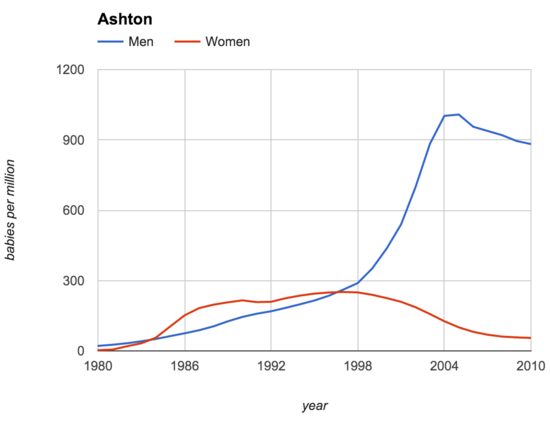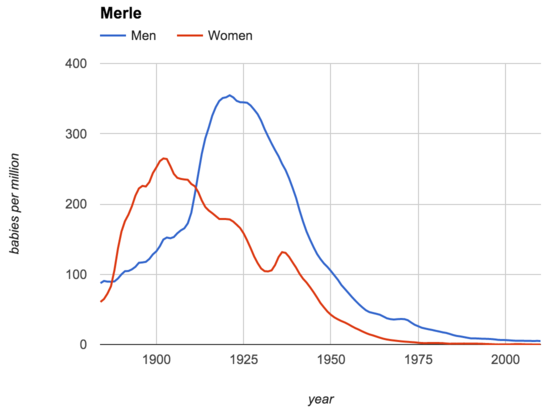And even some that have switched more than once: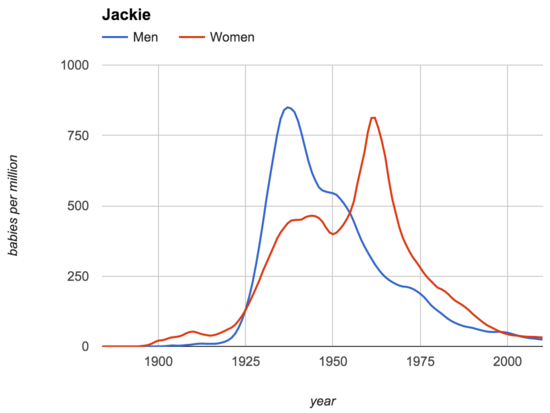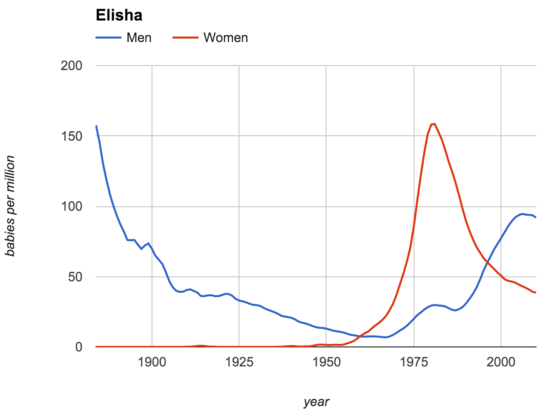One thing that jumps out at me about the female-to-male list, though, is that there are a lot of diminutives, like "Frankie".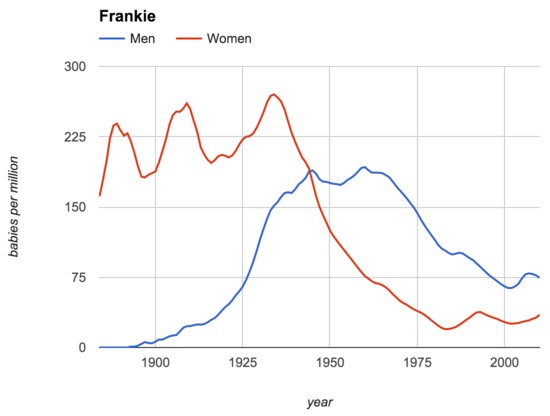My guess is what's happening here is that instead of a traditionally female name becoming a common male name, we're mostly seeing changing norms around what to put down as official names for Social Security purposes. The idea is that maybe there have always been more men going by "Frankie," but initially most of these men had been given the name "Frank" and later more parents started putting down the diminutive as the official name. I don't know how I'd test this guess though.

### Recent posts on blogs I like:

#### What are the results of more parental supervision and less outdoor play?

Ups and downs for mental health and injury rates The post What are the results of more parental supervision and less outdoor play? appeared first on Otherwise.

via Otherwise November 24, 2023